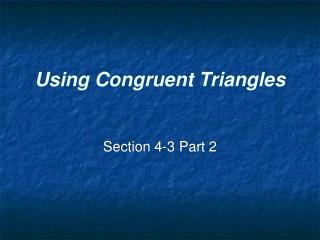DownloadDownload PresentationUsing Congruent Triangles

# Using Congruent Triangles

Download Presentation## Using Congruent Triangles

- - - - - - - - - - - - - - - - - - - - - - - - - - - E N D - - - - - - - - - - - - - - - - - - - - - - - - - - -
##### Presentation Transcript

1. Using Congruent Triangles Section 4-3 Part 2

2. Helpful Information • Deducing information about segments and angles AFTER proving that the two triangles are congruent. • Sometimes it will be helpful to plan the proof before you get started. • Remember to start with the 3-2-1. • CPCTC always follows 2 congruent triangles. • Start at the ends and fill in the middle last.

3. Complete the following proof:

4. Complete the following proof:

5. Complete the following proof:

6. Complete the following proof:

7. Complete the following proof:

8. Complete the following proof: